Home > CCAA8 > Chapter cca1 > Lesson cca1.1.2 > Problem1-15

1-15.

Finding and using a pattern is an important problem-solving skill you will use in algebra. The patterns in Diamond Problems will be used later in the course to solve other types of algebraic problems. Homework Help ✎

Look for a pattern in the first three diamonds below. For the fourth diamond, explain how you could find the missing numbers (?) if you know the two numbers (#).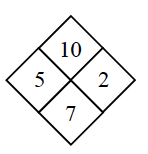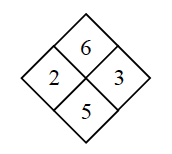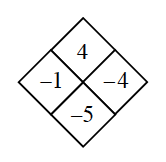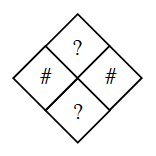Copy the Diamond Problems below onto your paper. Then use the pattern you discovered to complete each one.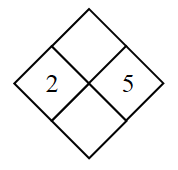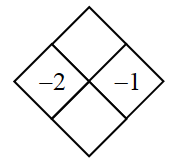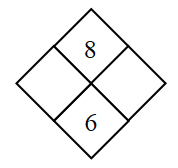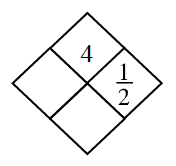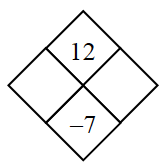What do you do to each of the # numbers to get to the ? numbers?

See the diamonds below. In the example diamonds, notice how one row added
together equals the bottom number, while that row multiplied equals the top number.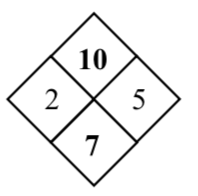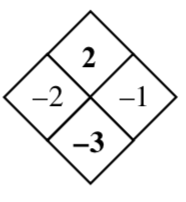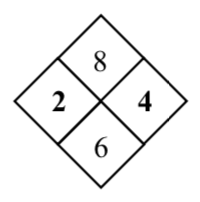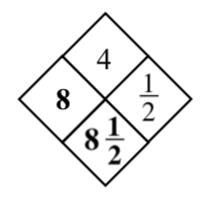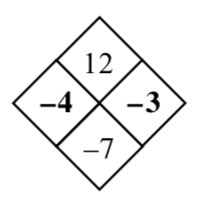In the example diamonds, notice how one row added together equals the bottom number, while that same row multiplied equals the top number.